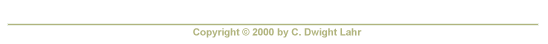## Select a video

(Clicking on a video camera image will start a video clip that uses RealPlayer.
If you do not already have it installed, you can get a free RealPlayer 8 Basic.)

# Principles of Calculus Modeling An Interactive Approach

## 1 Modeling Discrete Data

1.1 Introduction to the Issues

1.2 Lines in the Plane

1.3 Functions and Their Graphs

Is f(x) even, odd, or neither?

1.4 Defining New Functions from Old

1.5 Trigonometric Functions

1.6 Exponential and Logarithm Functions

1.7 Case Study: Modeling with Elementary Functions

## 2 Modeling Rates of Change

2.1 Introduction to the Issues

2.2 The Legacy of Galileo and Newton

2.3 Limits of Functions

With G(x) a piecewise defined, bumpy function:

Evaluate these limits:

2.4 Limits at Infinity

2.5 Continuity

Describe continuity of:

2.6 Tangent Lines and Their Slopes

2.7 The Derivative

2.8 Differentiation Rules

2.9 Derivatives of the Trigonometric Functions

2.10 The Mean Value Theorem

2.11 Implicit Differentiation

2.12 Derivatives of Exponential and Logarithm Functions

2.13 Newton's Method

2.14 Linear Approximations

2.15 Antiderivatives and Initial Value Problems

2.16 Velocity and Acceleration

2.17 Related Rates

2.18 Case Study: Torricelli's Law

## 3 Modeling with Differential Equations

3.1 Introduction to the Issues

3.2 Exponential Growth and Decay

3.3 Separable Differential Equations

3.4 Slope Fields and Euler's Method

3.5 Issues in Curve Sketching

3.6 Optimization

3.7 Case Study: Population Modeling

## 4 Modeling Accumulations

4.1 Introduction to the Issues

4.2 The Definite Integral

4.3 Properties of the Definite Integral

4.4 The Fundamental Theorem of Calculus

4.5 Techniques of Integration

4.6 Trapezoid Rule

4.7 Areas Between Curves

4.8 Volumes of Solids of Revolution

4.9 Arc Length

4.10 Inverse Trigonometric Functions

4.11 Case Study: Flood Watch

## 5 Culminating Experience

5.1 Case Study: Sleuthing Galileo